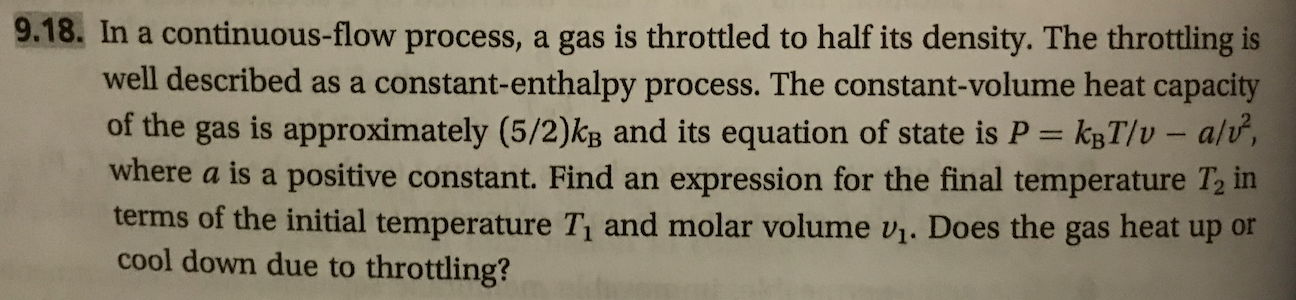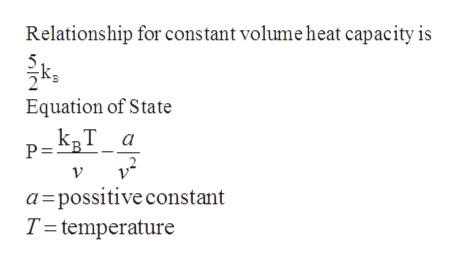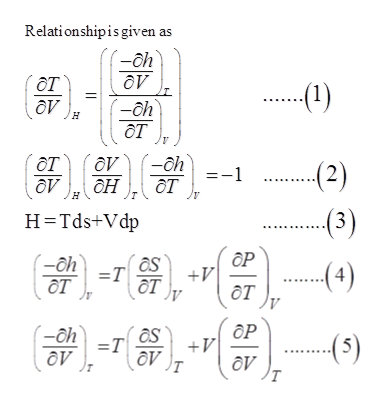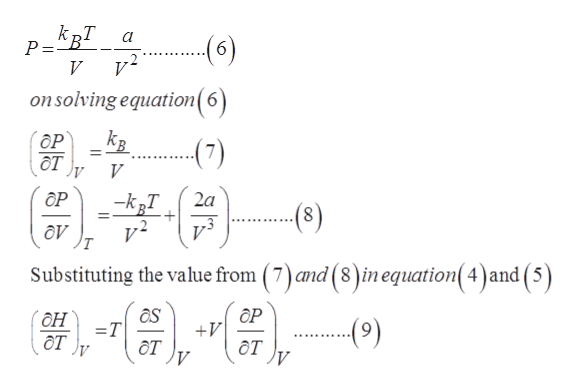# 9.18. In a continuous-flow process, a gas is throttled to half its density. The throttling iswell described as a constant-enthalpy process. The constant-volume heat capacityof the gas is approximately (5/2)kg and its equation of state is P kgT/v - alv,where a is a positive constant. Find anterms of the initial temperature T and molar volume v1. Does the gas heat up orcool down due to throttling?expression for the final temperature T2 in

Question
189 viewshelp_outlineImage Transcriptionclose9.18. In a continuous-flow process, a gas is throttled to half its density. The throttling is well described as a constant-enthalpy process. The constant-volume heat capacity of the gas is approximately (5/2)kg and its equation of state is P kgT/v - alv, where a is a positive constant. Find an terms of the initial temperature T and molar volume v1. Does the gas heat up or cool down due to throttling? expression for the final temperature T2 in fullscreen
check_circle

Step 1

Throttling process is defined as the process for which enthalpy remains constant that is no work is done and process will be in adiabatic condition

A process is considered for continuous flow process gas is throttling to half its densityhelp_outlineImage TranscriptioncloseRelationship for constant volume heat capacity is Equation of State kвТ_а P V a possitive constant T temperature fullscreen
Step 2

enthalpy of system is written as

If H is the enthalpy of system

From equation 3help_outlineImage TranscriptioncloseRelationshipis given as ду .-(1) (-ah от ду H (2) ду от ат ду дн H Tds+Vdp ОР as (oh=1T. +V ..4) OT V ОР chTS +V av дV T fullscreen
Step 3

From given relation

change in enthalpy ...help_outlineImage TranscriptioncloseP="B V (6) on solving equation( 6) ОР от (7) V OP kT 2a (8) (7)and (8)in equation(4) and (5) Substituting the value from он От )у as ОР (9) V =T д дт fullscreen

### Want to see the full answer?

See Solution

#### Want to see this answer and more?

Solutions are written by subject experts who are available 24/7. Questions are typically answered within 1 hour.*

See Solution
*Response times may vary by subject and question.
Tagged in

### Chemical Engineering